## Saturday, August 22, 2009

### Mathematics - Matrices (Part 1)

The question:
Write the matrix equation representing the following simultaneous equations and hence solve the equation 5y = 39 - 2x and 11x = 1 + 3y using matrices.Solution:
(Explanation can be obtained from the Properties of Matrices, presented after the answer).

Rewriting the simultaneous equations,
2x + 5y = 39
11x - 3 y = 1

The simultaneous equations may be represented by the matrix equation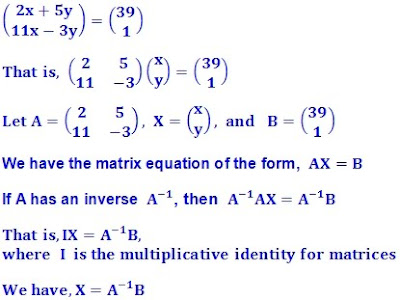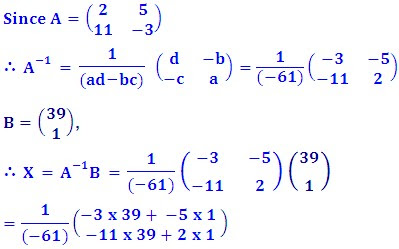Therefore x = 2, y = 7 (Answer).Picture is obtained from http://www.philipmantione.com/matrix1.jpg

Properties of Matrices

From:
http://www.sosmath.com/matrix/matrix0/matrix0.html - Introduction and Basic Operations (Author: M.A. Khamsi)

• Matrices involved in the addition operation must have the same size

The following are obtained from
Additional Mathematics, 8th Edition, by The Keng Seng, Loh Cheng Yee, Consultant: Dr. Yeap Ban Har, Shinglee Publishers Pte Ltd, Chapter 8 - “Matrix and its Applications”, Page 215 to 229.

Multiplication of Matrices:
• A matrix of the order m x n multiplied by a matrix of order n x p will result in a matrix of order m x p.

Given thatThe order of matrix A is 1 x 2 (1 row by 2 columns), of matrix B is 2 x 1 and of matrix C is 2 x 2.

For the matrix product AB to exist, the number of columns of matrix A must be equal to the number of rows of matrix B. The order of AB will be given by the number of rows of A and the number of columns of B.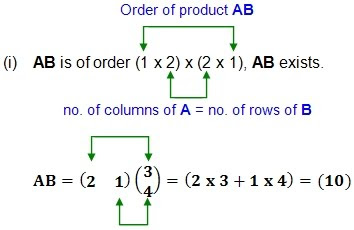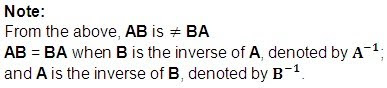The Inverse Matrix
In the real number system, there exists an additive identity, 0, such that a + 0 = 0 + a = a.

For matrix there also exists an additive identity which is the null matrix, 0, such that A + 0 = 0 + A = A.

A Null matrix is a matrix in which all the elements are equal to 0.considering only 2 x 2 matrices, we have:In the real number system, there exists an multiplicative identity, 1, such that a x 1 = 1 x a = a.

Similarly there exists for matrices, the multiplicative identity, I, such that AI = IA = A.

In the number system, for every number a, there is an additive inverse, -a, such that a + (-a) = 0;
and a multiplicative inverse
,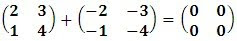The additive inverse of a matrix can be found easily.

The multiplicative inverses of matrices are the following.When the inverse of a matrix is mentioned, it generally refers to its multiplicative inverse.

If A is the inverse of B, then the order of A and B are the same, eg. 2 x 2.where the difference of the product of the elements on the leading diagonal and the product of the elements of the other diagonal is 2.

Pre-multiplying A by B, i.e. BA,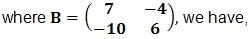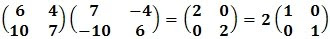Post-multiplying A by B, i.e. AB, we have,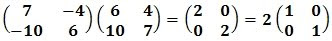The difference of the products of the elements in the leading diagonal and the other diagonal in A is 2, and the product AB is twice the unit matrix.General Cases:
Consider the general 2 x 2 matrixUsing the multiplicative inverse matrix rule above, let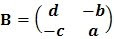In general,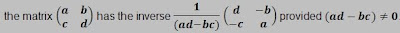The expression ad - bc is known as the determinant of the matrix A and is denoted by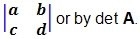If det A = 0, then the inverse of A is not defined becauseis not defined.

In such a case, A does not have an inverse.
Where a matrix does not possess an inverse, it is known as a singular matrix.

References